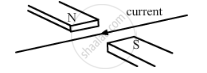Share

# A Horizontal Wire Carries a Current as Shown in Figure Below Between Magnetic Poles N and S: - CBSE Class 10 - Science

#### Question

A horizontal wire carries a current as shown in Figure below between magnetic poles N and S:Is the direction of the force on the wire due to the magnet:

(a) in the direction the current
(b) vertically downwards
(c) opposite to the current direction
(d) vertically upwards

#### Solution

vertically upwards
Applying Fleming's left-hand rule, we find that the force on the wire will act vertically upward.

Is there an error in this question or solution?

#### APPEARS IN

Lakhmir Singh Solution for Physics for Class 10 (2019 Exam) (2018 to Current)
Chapter 2: Magnetic Effects of Electric Current
Q: 30 | Page no. 93
Solution A Horizontal Wire Carries a Current as Shown in Figure Below Between Magnetic Poles N and S: Concept: Fleming’s Left Hand Rule.
S